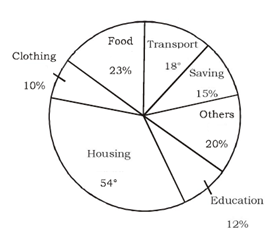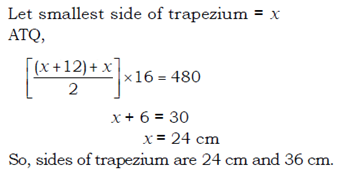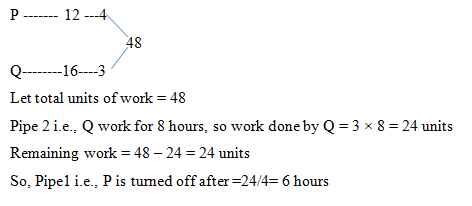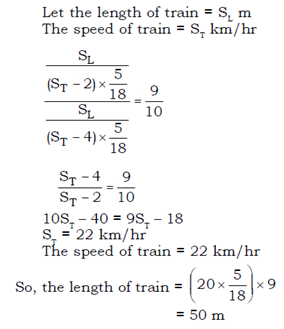# SSC CGL 2018 Practice Test Papers | Quantitative Aptitude (Day-32)

Dear Aspirants, Here we have given the Important SSC CGL Exam 2018 Practice Test Papers. Candidates those who are preparing for SSC CGL 2018 can practice these questions to get more confidence to Crack SSC CGL 2018 Examination.

[WpProQuiz 3227]

Click “Start Quiz” to attend these Questions and view Explanation

Directions: (1-5) The circle graph given below show the spending of a family on various items and its saving during a year. Study the graph and answer the following questions.1) If the total income of the family is Rs.80000,the expenditure on Education was _______

a) Rs. 8600

b) Rs. 9600

c) Rs. 8400

d) Rs. 7700

2) What percent of the total income was spent on Transport and other items?

a) 25%

b) 20%

c) 30%

d) 32%

3)  Out of the total income of Rs.80000, the difference of the expenses on Housing and Transport was ___________

a) Rs. 6400

b) Rs. 7200

c) Rs. 8000

d) Rs. 9600

4) What is the ratio of amount spent on Clothing and other items?

a) 1:2

b) 1:1

c) 2:1

d) 1:3

5) If the family saved Rs.60000/year What is the expenditure amount/ year?

a) 300000

b) 320000

c) 340000

d) None of these

6) The area of a trapezium is 480 cm2. If the difference between its parallel sides is 12 cm and the distance between them is 16 cm, then the parallel sides are –

a) 12 cm and 24 cm

b) 16 cm and 28 cm

c) 20 cm and 32 cm

d) 24 cm and 36 cm

7) A man sells a car at 10% loss to his friend. If his friend sells the car for Rs.54000 and gets 20% profit on it, what was the actual price of the car?

a) Rs. 48000

b) Rs. 50000

c) Rs. 60000

d) Rs. 73500

8) Two pipes P and Q can fill a tank in 12 hours and 16 hours respectively. If both pipes are opened together, find when the first pipe must be turned off so that the tank may be just filled in 8 hours.

a) 4 hours

b) 5 hours

c) 6 hours

d) 3 hours

9) A train overtakes two persons, who arewalking in the same directions in which the train is running at the rate of 2 km/hr and 4 km/hr and passes them completely in 9 and 10 sec respectively. What is the length of the train ?

a) 72 m

b) 60 m

c) 54 m

d) 50 m

10) A right circular cone is cut by a plane parallel to its base in such a way that the slant heights of the original and the smaller cone thus obtained are in the ratio 2 : 1. If V1 and V2 are respectively the volumes of the original cone and of the new cone then what is V1 : V2 ?

a) 2 : 1

b) 3 : 1

c) 4 : 1

d) 8 : 1

Expenditure on education = 80000 ×12/100= Rs. 9600

Percentage spent on transport =18/360× 100= 5%

Percentage spent on other items = 20%

Required percentage = (20 + 5)% = 25%

Required amount =(54-18)/360× 80000= Rs. 8000

amount spent on Clothing=10%

amount spent on Others=20%

Ratio=10:20=1:2

Amount Spent =60000/15×85=340000Cost price for car for friend = 54000 ×100120= Rs. 45000

So, cost price for Man = 45000 ×100/90 = Rs. 50000